### Home > CALC > Chapter 12 > Lesson 12.3.1 > Problem12-111

12-111.
1. The function f has derivatives of all orders within its radius of convergence of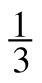about x = 0 and its Maclaurin series is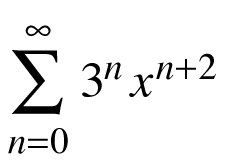. Homework Help ✎

1. Write the first four derivatives of f .

2. Expand the Maclaurin series of f out to four terms. Then find the integral of this polynomial, p4(x).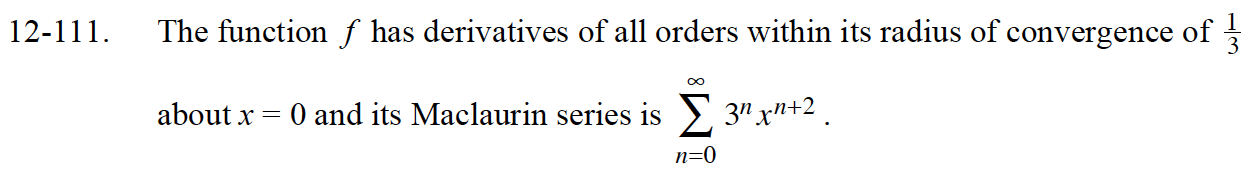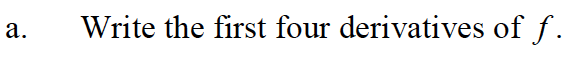Remember, 3n is just a number, so: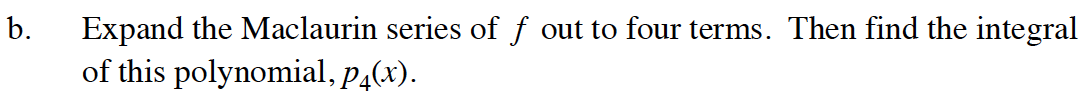$p_4(x)=3^0x^{0+2}+3^1x^{1+2}+3^2x^{2+2}+3^3x^{3+2}$